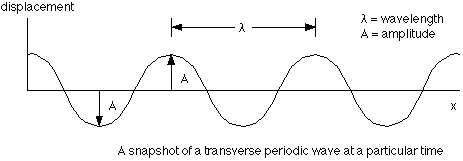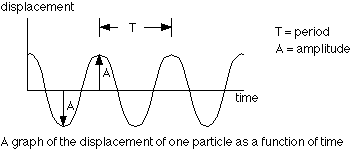## Waves

3-1-99

Sections 11.7 - 11.9

We'll shift gears now, spending some time on waves.

### Types of waves

A wave is a disturbance that transfers energy from one place to another without requiring any net flow of mass. For now, we'll focus on mechanical waves, requiring a medium in which to travel. Light, and other electromagnetic waves, do not require a medium; we'll deal with those later in the semester.

Waves can be broadly separated into pulses and periodic waves. A pulse is a single disturbance while a periodic wave is a continually oscillating motion. There is a close connection between simple harmonic motion and periodic waves; in most periodic waves, the particles in the medium experience simple harmonic motion.

Waves can also be separated into transverse and longitudinal waves. In a transverse wave, the motion of the particles of the medium is at right angles (i.e., transverse) to the direction the wave moves. In a longitudinal wave, such as a sound wave, the particles oscillate along the direction of motion of the wave.

Surface waves, such as water waves, are generally a combination of a transverse and a longitudinal wave. The particles on the surface of the water travel in circular paths as a wave moves across the surface.

### Periodic waves

A periodic wave generally follows a sine wave pattern, as shown in the diagram.A number of parameters can be defined to describe a periodic wave:

wavelength - the length of one cycle
amplitude - the maximum distance a particle gets from its undisturbed position
period (T) - time required for one cycle
frequency (f = 1/T) - number of cycles in a certain time, usually in 1 second.
speed - this is given by v = frequency x wavelengthNote that the frequency of the wave is set by whatever is producing the disturbance, while the speed is determined by the properties of the medium. The wavelength can then be found from the equation above.

A sine wave oscillation is also followed by each particle in the medium, as the following diagram shows:There is a big difference between what the wave does and what the particles in the medium do. As the wave travels through the medium, the particles of the medium oscillate in response to the wave. In a uniform medium, the wave travels at constant speed; each particle, however, has a speed that is constantly changing. The maximum speed of a particle is determined by the wave amplitude and frequency.

### The speed of a wave

The speed of a wave traveling through a medium depends on properties of the medium. For a wave to propagate, each disturbed particle must exert a force on its neighbor. The wave speed is essentially the speed at which the neighboring particle responds to this force; that response time is determined by the mass of the particle and the size of the force exerted. For a transverse wave on a rope or stretched spring, the wave speed is determined by the tension (F) and the mass per unit length:For a sound wave, the speed is determined by either Young's modulus or the bulk modulus (discussed in chapter 9) and the density: